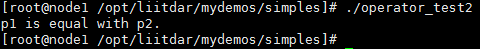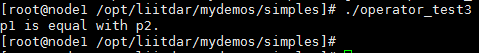# 1. 概述

## 1.1 what

operator 是C++的一个关键字，它和运算符（如=）一起使用，表示一个运算符重载函数，在理解时可将operator和运算符（如operator=）视为一个函数名。

• 使重载后的运算符的使用方法与重载前一致

• 扩展运算符的功能只能通过函数的方式实现（实际上，C++中各种“功能”都是由函数实现的）

## 1.3 how

• 将操作符重载实现为类的成员函数；

• 操作符重载实现为非类的成员函数（即全局函数）。

### 1.3.1 将操作符重载实现为类的成员函数

`    bool operator==(const person& ps)    {        if (this->age == ps.age)        {            return true;        }        return false;    }`

`#include <iostream>using namespace std;class person{private:int age;public:person(int nAge){this->age = nAge;}bool operator==(const person& ps){if (this->age == ps.age){return true;}return false;}};int main(){person p1(10);person p2(10);if (p1 == p2){cout << "p1 is equal with p2." << endl;}else{cout << "p1 is not equal with p2." << endl;}return 0;}`### 1.3.2 操作符重载实现为非类的成员函数（即全局函数）

`#include <iostream>using namespace std;class person{public:int age;};// 左操作数的类型必须被显式指定// 此处指定的类型为person类bool operator==(person const& p1 ,person const& p2){if (p1.age == p2.age){return true;}else{return false;}}int main(){person p1;person p2;p1.age = 18;p2.age = 18;if (p1 == p2){cout << "p1 is equal with p2." << endl;}else{cout << "p1 is NOT equal with p2." << endl;}return 0;}`### 1.3.4 操作符重载的方式选择

• 如果一个重载操作符是类成员，那么只有当与它一起使用的左操作数是该类的对象时，该操作符才会被调用；而如果该操作符的左操作数确定为其他的类型，则操作符必须被重载为全局函数；

• C++要求'='、'[]'、'()'、'->'操作符必须被定义为类的成员操作符，把这些操作符通过全局函数进行重载时会出现编译错误

• 如果有一个操作数是类类型（如string类），那么对于对称操作符（比如==操作符），最好通过全局函数的方式进行重载。

### 1.3.5 操作符重载的限制

• 重载后操作符的操作数至少有一个是用户定义类型；

• 不能违反原来操作数的语法规则；

• 不能创建新的操作符；

• 不能重载的操作符包括（以空格分隔）：sizeof . .* :: ?: RTTI类型运算符

• =、()、[]、以及 ->操作符只能被类的成员函数重载

### 1.3.6 操作符重载的详细用法

• 赋值运算符的重载函数（operator=），点击此处